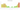# лв.

## Bulgarian Lev

#### how has the value of the currency changed in the last year?## USD 1 = BGN 1.8515

How much is10 US dollars worth inBulgarian leva?
At the current exchange rate, 10 US dollars is worth 18.51 Bulgarian leva
How much is50 US dollars worth inBulgarian leva?
At the current exchange rate, 50 US dollars is worth 92.57 Bulgarian leva
How much is100 US dollars worth inBulgarian leva?
At the current exchange rate, 100 US dollars is worth 185.15 Bulgarian leva
How much is500 US dollars worth inBulgarian leva?
At the current exchange rate, 500 US dollars is worth 925.73 Bulgarian leva
How much is2,000 US dollars worth inBulgarian leva?
At the current exchange rate, 2,000 US dollars is worth 3,702.9 Bulgarian leva
How much is10 US dollars worth inBulgarian leva?
At the current exchange rate, 10 US dollars is worth 18.51 Bulgarian leva
How much is50 US dollars worth inBulgarian leva?
At the current exchange rate, 50 US dollars is worth 92.57 Bulgarian leva
How much is100 US dollars worth inBulgarian leva?
At the current exchange rate, 100 US dollars is worth 185.15 Bulgarian leva
How much is500 US dollars worth inBulgarian leva?
At the current exchange rate, 500 US dollars is worth 925.73 Bulgarian leva
How much is2,000 US dollars worth inBulgarian leva?
At the current exchange rate, 2,000 US dollars is worth 3,702.9 Bulgarian leva